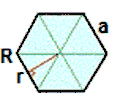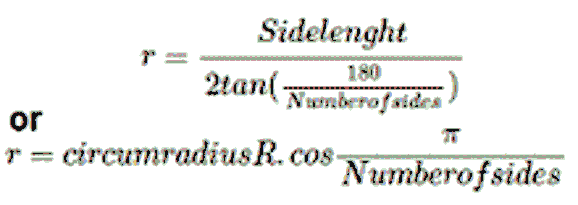# Apothem of a Regular Polygon Calculator

The calculator calculates the apothem of a regular polygon based on given values of the side length, circumradius and number of sides for selected type of polygon. User can select the unit as meter, centimeter, inches and feet.

 Select a polygon Select Trigon (Triangle) Tetragon (Quadrilateral) Pentagon Hexagon Heptagon Octagon Enneagon (nonagon) decagon hendecagon dodecagon tridecagon tetradecagon pentadecagon hexadecagon Other Enter Side Length or Circumradius Select Side Length(a) Circumradius(R) Enter the Side Length: (a) Select Unit meters centimeters feet inches Enter the Circumradius: (R) Select Unit meters centimeters feet inches Enter the number of sides: (n)

 Apothem of a regular polygon (r):

The word apothem can refer to the length of that line segment. The apothem of a regular polygon is a line segment from the center to the midpoint of one of its sides.

Analogically, it is the line drawn from the center of the polygon that is perpendicular to one of its sides.